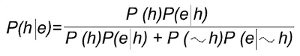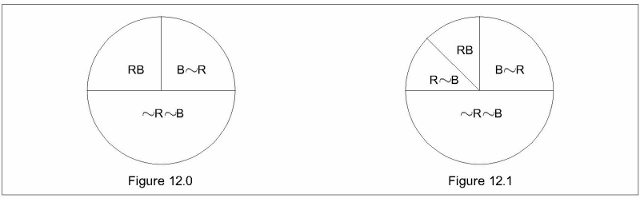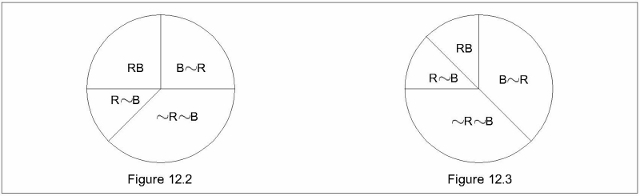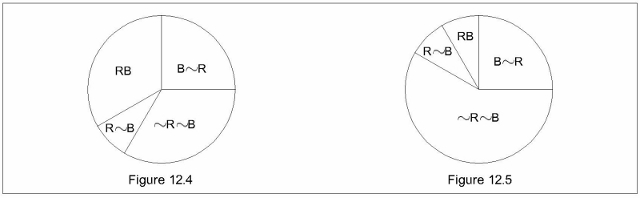CHAPTER 12

CONFIRMATION

12.1. I claim

(a) that what is nowadays considered the canonical Bayesian position on Hempel's paradox is incomplete, since it examines only one among many possible alternatives;

(b) that the analysis of the examined alternative and the consequent solution are misleading

(c) that there is an exhaustive solution whose conclusions comply punctually with our intuition.

Since, in order to report the canonical Bayesian position, I make reference to Howson and Urbach's work listed in Bibliography (from now on: H&U), I accept their notational conventions, although I dissent from a symbology where the probability is sometimes monadic (unconditional) and sometimes dyadic (conditional).

12.2. First of all, I define by

(12.i)      We,h = P(h|e) – P(h)

that is, in my symbology,

(12.i*)       Wk',k°,h = P(h|k°&k') – P(h|k°)

the confirmation value of evidence e on a hypothesis h, (the confirmation value of an acquirement k' on a hypothesis h, given a basic statute) and I say that e validates h (invalidates h; is uninfluential upon h), iff We,h>0 (iff We,h<0; iff We,h=0). So, according to (12.i), not only "to validate", but also "to invalidate" and "to be uninfluential" express values of confirmation (ranging from -1 to +1). In §13.5.2.1 a representation of confirmation values will be sketched.

12.3. Hempel's paradox is well known. Briefly. Nicod's postulate, applied to the classical example, states that a hypothesis like

(12.ii)       All Rs are B (all ravens are black)

is validated by evidence of something that is both R and B. Then, since the unexceptionable Principle of Equivalence states that any evidence has the same confirmation value on logically equivalent hypotheses, and since by Modus Tollens (12.ii) is logically equivalent to

(12.iii)       All ~Bs are ~R

evidence of a ~B~R (of a grey pistol GP, say) ought to validate (12.ii); a conclusion which indeed hurts our common sense.

12.4. The deep incompatibilities among the various theories of probability regard many aspects of the matter (the philosophical approach, the Principle of Indifference, the problem of inductive generalizations and so on); but, as far as I know, they do not regard the logical and mathematical structure. In particular no one denies the validity of Bayes's theorem, since it is formally derivable from universally accepted axioms. In this sense speaking of the Bayesian position seems to me rather misleading; how could we legitimate an anti-Bayesian position? In this sense, then, I think it would be better to speak of a strictly axiomatic approach.

12.4.1. Since Bayes's Theorem (H&U, p.21) states that

(12.iv)we can formally derive

P(h|e) > P(h) iff P(e|h) > (P(h)P(e|h) + P(~h)P(e|~h))

that is iff P(e|h) >(1- P(~h))P(e|h)) + P(~h)P(e|~h))

that is iff P(e|h) >P(e|h)- P(~h)P(e|h)) + P(~h)P(e|~h))

therefore

(12.v)      P(h|e) > P(h) iff P(e|h) > P(e|~h))

(obviously P(e|h) > P(e|~h)). implies P(~h)P(e|h)) > P(~h)P(e|~h)).

In our specific case (12.v) becomes

(12.vi)       P(h|RB) > P(h) iff P(RB|h) > P(RB|~h))

and the formal derivation above proves that (12.v) and (12.vi) are theorems, that is unobjectionable achievements. Furthermore, since analogous derivations can be re-proposed where ">" is replaced by "<" or by "=", we can conclude that evidence of a black raven validates (invalidates, is uninfluential upon) the hypothesis h that all ravens are black iff such evidence is more (less, equally) probable under h than under ~h. Yet Nicod's postulate omits the conditioning clause (in the case under scrutiny it reduces (12.vi) to its first member) and as such it is valid only in those contexts where the same conditioning clause is satisfied. And simply because, roughly, the assumption that observing a black raven is more probable if all ravens are black seems plausible (here is the second member of (12.vi)), Nicod's postulate, roughly, seems correct. Nevertheless, simply because discordant contexts (that is contexts where the second member of (12.vi) is not valid) are possible (although less plausible), Nicod's postulate cannot constitute a general rule for a systematic approach to the matter.

12.4.2. The general rule is inferred by (12.v) and runs as follows:

(12.vii)       Evidence of something that is both X and Y validates (invalidates, is uninfluential upon) the

hypothesis that all Xs are Y, iff the eventual presence of some X~Y decreases (increases, does

not change) the probability of observing a XY.

In its turn, as soon as we realize that it is not necessary to restrict the evidence to the observation of a XY, we can furtherly generalize (12.vii) in

(12.viii)       An evidence validates (invalidates, is uninfluential upon) the hypothesis that all Xs are Y

iff the eventual presence of some X~Y decreases (increases, does not change) the

probability of the same evidence

The fundamental point is that Nicod's postulate, (12.vii) and (12.viii) do not have the same rank: while the former is a questionable supplementary proposal, (12.vii) and (12.viii) are unquestionable achievements inferred by universally accepted axioms.

12.4.3. The inversion between the roles of h and e showed by (12.v) is momentous: a problem of confirmation can be solved without any collation between the probabilities of h ante and post the acquirement of e, since it is sufficient to collate the probability of e under h and the probability of e under ~h. Therefore it is explicitly and formally ascertained that every problem of confirmation concerns the collation between two incompatible universes which differ with respect to h or ~h. It is understood that I speak of two universes without any ontological implication. We have to remember the general scenery of reference. The universe we are living in is the only real universe; but as soon as we ask ourselves about the problem under scrutiny, we must compare two incompatible configurations and the probabilities of observing a black raven in each of them.

12.4.4. In empirical practice, the probability of observing a certain individual in our real universe depends on hundreds of parameters, yet, in order not to complicate the discourse with contingencies of no theoretical moment, I assume

- that a number is assigned to every individual of the universal population to which the reasoning is referred

- that the numerical output of an opportunely programmed computer indicates the observed individual

- that the outputs are equiprobable, so that the probability of observing an individual belonging to a certain set is directly proportional to the cardinality of the same set.

These assumptions are of no theoretical moment since Hempel's paradox can be exactly re-proposed even under them.

12.5. The intuitive understanding of my analysis can be helped by an easy representation where the various configurations are represented by circles partitioned in sectors: one circle for each configuration, one sector for each set of individuals. Let me emphasize that this representation is simply an adaptation of Eulerian circles (Venn's diagrams); contrary to our usual ®, it is intrinsically extensional. Yet, since for the moment I am mainly interested in the clearness of the diagrams and of their collations, the areas of the various sectors have a merely qualitative course; the only areal dependences are

- an empty set is represented by a null sector

- although the area of a sector increases (decreases) with the cardinality of the represented set, no direct proportionality exists between areas and cardinalities.

So for instance the RB-sector of Figure 12.0is greater by far than the quantitatively correct one (black ravens are not a quarter of all the individuals).

Figure 12.0 represents the basic configuration Ω°, that is the h–compatible configuration where all ravens are black (no R~B-sector). Of course there are many different ~h-compatible configurations. In some of them the RB-set is smaller than in Ω° (the sector representing such a set is smaller than in Figure 12.0), and as such evidence of a black raven validates h. In others the RB-set is greater than in Ω° (…) and as such evidence of a black raven invalidates h. In the remainder the RB-sector is the same of Ω° (…) and as such evidence of a black raven is uninfluential upon h. Therefore, since a problem of confirmation is represented by the comparison between two figures, the specification of the ~h-compatible configuration to be collated with Ω° is indispensable.

12.5.1. Once assumed that Ω° is the basic configuration where

(12.ix)      All ravens are black

the privileged configuration where

(12.x)      Not all ravens are black

is suggested by the same formulation of (12.ix) and (12.x); in fact the opposition between the "all" of (12.ix) and the "not all" of (12.x) suggests the reference to a same domain of quantification. Therefore we are induced to think of a common set of ravens without involving other (and not even named) categories of individuals. In other words, under their most spontaneous interpretation (12.ix) and (12.x) propose a dilemma whose empirical solution consists in examining the colour of any raven belonging to a previously given set. Figure 12.1 represents just this configuration Ω1 which differs from Ω° only because in Ω1 the ravens-sector of W° is now partitioned in an RB sector and in an R~B–sector.

Under such an interpretation, since in Figure 12.0 (where h) the RB-sector is greater than in Figure 12.1 (where ~h), evidence of a black raven validates h.

12.5.2. If all ravens are black, all non-black individuals are non-ravens (Modus Tollens). Therefore also

(12.xi)       All non-black individuals are non-ravens

is true under Ω° (actually Figure 12.0 respects (12.xi)). Nevertheless as soon as the considerations proposed in §12.5.1 are applied to the opposition between (12.xi) and

(12.xii)       Not all non-black individuals are non-ravens

we realize that, contrary to (12.ix) and (12.x) where "all" and "not all" refer to the set of ravens, in (12.xi) and (12.xii) "all" and "not all" refer to the set of non-black individuals and that, therefore, the most spontaneous interpretation leads to the configuration Ω2 represented in Figure 12.2that is the configuration which differs from Ω° because some non-black raven takes the place of some non-black non-raven. In other words, under their most spontaneous interpretation, (12.xi) and (12.xii) propose a dilemma whose empirical solution consists in examining a previously given set of non-black individuals in order to ascertain if any among them is a raven.

The figures make immediately evident that while Nicod's criterion in the formulation (12.ii) is valid with reference to (Ω°, Ω1) for the number of black ravens (the area of the RB-sector) is greater in Ω° than in Ω1, the same criterion is not valid with reference to (Ω°, Ω2) for the number of black ravens (the area of the RB-sector) is the same in Ω° and in Ω2. Reciprocally, while Nicod's criterion in the formulation (12.iii) is valid with reference to (Ω°, Ω2), it is not valid with reference to (Ω°, Ω1).

On the contrary (12.vii) and (12.viii) are always valid.

12.5.3. Incidentally. While (12.ix) and (12.x) are introduced by merely emphasizing new lines, (12.xi) and (12.xii) are introduced by hyperlinguistic new lines (… is true under Ω°…); furthermore they all are metalinguistically recycled (… the "all" …).

12.6. As for our problem (let me reason directly on the representation), the numerous diagrams Ωi resulting from the various tetrapartitions of a circle in the four sectors representing BR, B~R, R~B and ~R~B, can be classified in compliance with their respective confirmation values, that is in compliance with the areal ratios of homologous sectors in Ω° and in Ωj. So, for instance, an easy collation between Figure 12.0 and Figure 12.3 shows that the latter represents a configuration where

P(RB|h) > P(RB|~h)

P(R~B|h) < P(R~B|~h)

(in particular P(R|h) = P(R|~h))

P(~R~B|h) > P(~R~B|~h)

P(B~R|h) < P(B~R|~h)

(in particular P(~B|h) = P(~B|~h)).

Analogously Figure 12.4represents a configuration where

P(RB|h) < P(RB|~h)

P(R~B|h) < P(R~B|~h) (therefore P(R|h) < P(R|~h))

P(~R~B|h) > P(~R~B|~h)

P(B~R|h) = P(B~R|~h) (therefore P(~B|h) < P(~B|~h))

Figure 12.5 represents a configuration where

P(RB|h) > P(RB|~h)

P(R~B|h) < P(R~B|~h)

(in particular P(R|h) > P(R|~h))

P(~R~B|h) < P(~R~B|~h)

P(B~R|h) = P(B~R|~h)

and so on.

12.7. In order to connect my analysis with the solution proposed by Howson and Urbach I need to transform unconditional probabilities into conditional ones and vice versa. This task is accomplished by the equivalence

(12.xiii)

(P(R|h) = P(R)) iff (P(R|h) = P(R|~h))

whose formal proof follows:

P(R|h) = P(R) (protasis)

P(R|h) = P(R&h)/P(h) (definition of conditional probability; H&U (4) p.14)

P(R&h) = P(R)P(h) (from protasis and definition)

P(R&h) = P(R)P(h|R) (well known theorem)

P(R&~h) = P(R)P(~h|R) (idem)

P(R&h) + P(R&~h) = P(R) (since (P(~h) = 1- P(h))

P(R)P(h) = P(R) - P(R&~h)

P(R)(1-P(h)) = P(R&~h)

P(R)P(~h) = P(R&~h)

P(R)=P(R&~h)/P(~h)=P(R|~h) (definition of conditional probability)

therefore

if P(R|h) = P(R) then P(R|h) = P(R|~h).

Reciprocally

P(R|h) = P(R|~h) (protasis)

(P(R&~h) / P(~h)) = (P(R&h) / P(h))

(definition of conditional probability)

P(R&~h)P(h) = P(R&h)(1- P(h))

(P(~h) = 1- P(h))

P(R&h) = P(R&~h)P(h) + (P(R&h)P(h) = P(R)P(h)

P(R) = (P(R&h)/ P(h) = (P(R|h) (definition of conditional probability)

therefore

if (P(R|h) = P(R|~h)) then (P(R|h) = P(R)).

12.8. On the basis of (12.xiii), to assume, as Howson and Urbach do (last lines of p.100),

(12.xiv)      P(R|h) = P(R)

means to assume

(12.xv)      P(R|h) = P(R|~h)

therefore (I continue reasoning directly on the representation) it means to admit only configurations where the R–sector (as in Figure 12.1 and 12.3) is the same of Figure 12.0; in fact (12.xiv) and (12.xv) are contradicted by configurations where (as in Figure 12.2 and 12.4 and 12.5) the R–sector is different from Figure 12.0.

12.8.1. Yet, besides (12.xiv), Howson and Urbach (second line of p. 101: By parallel reasoning... ) assume

(12.xvi)      P(~B|h)=P(~B)

so that

(12.xvii)       (P(R|h)=P(R)) & P(~B|h)=P(~B)

is their total assumption. This means that the ~h-configuration they oppose to Ω° is Ω3 that is the configuration represented in Figure 12.3, where

- the R~B–sector is non-null, thus complying with ~h

- the area of both the R-sector and the ~B-sector are the same as Ω°, thus complying with (12.xvii).

In Ω3 both the observation of a black raven and of a non-black non-raven validate h , as both the RB–sector and the (~R~B)–sector are greater in Figure 12.0 than in Figure 12.3.

The canonical Bayesian solution accepts such a conclusion and claims it is not at all paradoxical because, since confirmation is a matter of degree (H&U, p.100), as soon as we give the raven sector its nearly infinitesimal area we realize that the h-validation by a ~R~B–observation (diagrammatically: the difference between the areas of the two ~R~B-sectors) is of so low a degree as to be intuitively imperceptible.

12.9. I agree with the claim that confirmation is a matter of degree. yet I disagree radically with the claim that the solution of Hempel's paradox is a matter of degree. No doubt that the ratio 1/N between ravens and individuals of the universe is very little, but anyhow it is not zero, since ravens do exist. Therefore MN observations of non-black non-ravens and M observations of black ravens ought to validate h at the same degree. Which is not the case. The discovery of a huge mafia armoury crammed with 1000N grey pistols, would be a hard stroke to organized crime, but it would not be a validation of h, neither at a lowest degree; also because, if it were, it would also be a validation of the hypothesis that all dolphins are trifling or that all Messalina's lovers were brown-haired and so on.

These considerations induce me to think firmly that the path leading to the very solution of Hempel's paradox is not the standard Bayesian one.

12.10. In order to expose plainly what seems to me the right path, let me imagine an ornithological phenomenon entailing a configuration where (12.xvii) is satisfied. Some mutation in the genoma of ravens resulted in a new and very aggressive breed of black-and-white ravens; this phenomenon determined a chromatic disturbance in the neighbouring species such that for every raven proceeding from black to black-and-white, a magpie proceeded from black-and-white to black. The plausibility of this phenomenon is scarce, nevertheless its scarce plausibility, far from weakening my argument, strengthens it. In fact the solution I claim is based on the following manifest points:

a) the set of non-black non-ravens is extremely heterogeneous, since it includes pink pillows, green apples, white freezers, yellow geishas, grey pistols, blue hand-bombs, black-and-white magpies et cetera;

b) in order to satisfy (12.xvii) we must renounce the most plausible way out (that is Ω1) according to which the apparition of non-black ravens is an ornithological phenomenon confined to the set of ravens;

c) renouncing Ω1 does not at all mean renouncing the conviction that the apparition of non-black ravens is an ornithological phenomenon: simply we think that the apparition in Ω3 of non-black ravens, instead of being an ornithological phenomenon confined to the set of ravens as in Ω1, is an ornithological phenomenon affecting also individuals which are not ravens;

d) under this condition, the most plausible belief is that the non-ravens affected by an ornithological phenomenon born in the set of ravens are individuals of the neighbouring species, since it would be grotesque to claim that a mutation in the genoma of ravens determines a magic blackening of some grey pistols or pink pillows et cetera;

e) if the grey pistols subset is not affected by the eventual appearance of non-black ravens, evidence of a grey pistol is uninfluential upon the hypothesis that all ravens are black.

12.10.1. Diagrammatically the extreme heterogeneity of the non-black non-ravens set is represented by a partition of the ~R~B–sector in as many subsectors as the specific subsets. And d) tells us that the GP-subsector representing the grey pistols of Ω3 is the same as that of Ω°, therefore that the observation of a grey pistol is uninfluential upon the hypothesis that all ravens are black (so legitimating also under Ω3 my position about the mafia armoury).

12.10.2. Symbolically. I recognize that if we accept (12.xvii) setting aside any consideration of plausibility,

(12.xviii)      P(~R~B|h) > P(~R~B|~h)

is unobjectionably valid; nevertheless (12.xviii), far from entailing the grotesque

(12.xix)       P(GP|h) > P(GP|~h)

strongly suggests

P(GP|h) = P(GP|~h)

that is, on the basis of (12.v) and (12.xiii),

(12.xx)       P(h|GP) = P(h)

and (12.xx), through (12.i), implies WGP,h = 0.

12.10.3. My solution also explains why, under (12.xvii), ascertaining that the black-and-white vest over yonder is a football jersey provides us with a piece of information uninfluential upon h, while ascertaining that the black-and-white bird over yonder is a magpie provides us with a piece of information validating h.

12.10.4. Let me imagine a universe whose members are only ravens, magpies and pistols. The argument based on the degree of confirmation would fail, but the paradoxicality of the conclusion would remain. Another good reason for rejecting the standard Bayesian solution.

12,11. A final step is necessary to overcome any eventual suspicion of residuary paradoxicality. Since a grotesque claim is not a self-contradictory claim, somebody might refuse to renounce (12.xix), thus legitimating a configuration where undoubtedly evidence of a grey pistol validates the hypothesis that all ravens are black. Would then the paradox revive? Not at all. A paradox arises only when its disconcerting conclusion ensues from non-disconcerting premises. And if someone is so credulous to believe (or so arrogant to pretend) that a mutation in the chromatic gene of some raven also entails a counterbalancing chromatic modification in the metallurgy of some grey pistol, then he cannot consider as a paradoxical result that evidence of a grey pistol validates h. Such a conclusion is simply the due consequence of the disconcerting premise (since under (12.xix) there are more grey pistols in Ω° than in Ω3, the probability of observing a grey pistol is greater in the configuration where all ravens are black).

12.12. Until now, in order to follow the standard Bayesian solution, I have reasoned under (12.xvii). Yet the extrapolation of the analysis to configurations where

(P(R|h) ≠ P(R|~h))

or where

(P(~B|h)≠ P(~B|~h)

does not present any difficulty. For instance Figure 12.4 represents a configuration (Ω4) where

(P(R|h)<P(R|~h))&(P(~B|h)>P(~B|~h))

(Ω4 can be justified by supposing that the mentioned very aggressive breed of black-and-white ravens carried out a massacre of the neighbouring non-black species, so promoting a proliferation of black ravens). Analogously Figure 12.5 represents a configuration (Ω5) where

(12.xxi)      (P(R|h) > P(R|~h)) & (P(~B|h) < P(~B|~h))

(Ω5 can be justified by supposing that the aggressivity of black-and-white ravens provoked so violent a raven-phobic reaction of the neighbouring non-black species that both breeds of ravens are at the risk of extintion while the non-black neighbouring species are proliferating). And so on.

In this sense my approach, grounded on

- the substitution of (12.vi) or (12.vii) to Nicod's postulate

- the partition of the ~R~B–set in distinct subsets (categories)

- the disconcerting unreasonableness of the hypothesis according to which a chromatic mutation in some ravens ought to determine a change in the colour of individuals belonging to absolutely far categories (as for instance grey pistols)

- the non-paradoxicality of a disconcerting conclusion inferred by disconcerting premises,

allows a systematic analysis whose results are in full accordance with our intuitive requirements.

For instance, let me take two lines to discuss (12.xxi).      Since black ravens are very rare in Ω5, evidence of a black raven validates h. On the contrary evidence of a non-black non-raven, owing to the proliferation of the non-black neighbouring species, validates ~h if the observed individual belongs to a proliferating species (a magpie, say), while it is uninfluential if the observed individual belongs to an unaffected ornithological species (a golden eagle, say) or even more if the observed individual belongs to a totally heterogeneous category of individuals (a grey pistol, say).